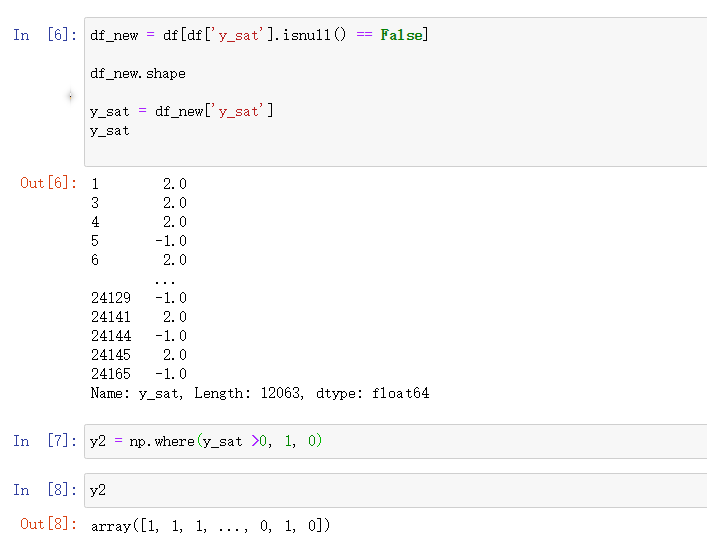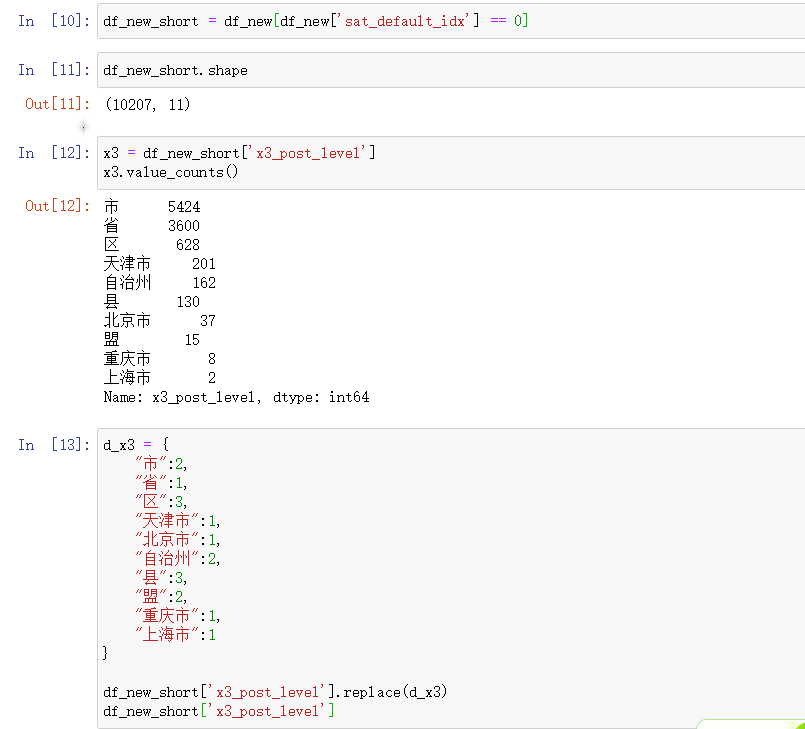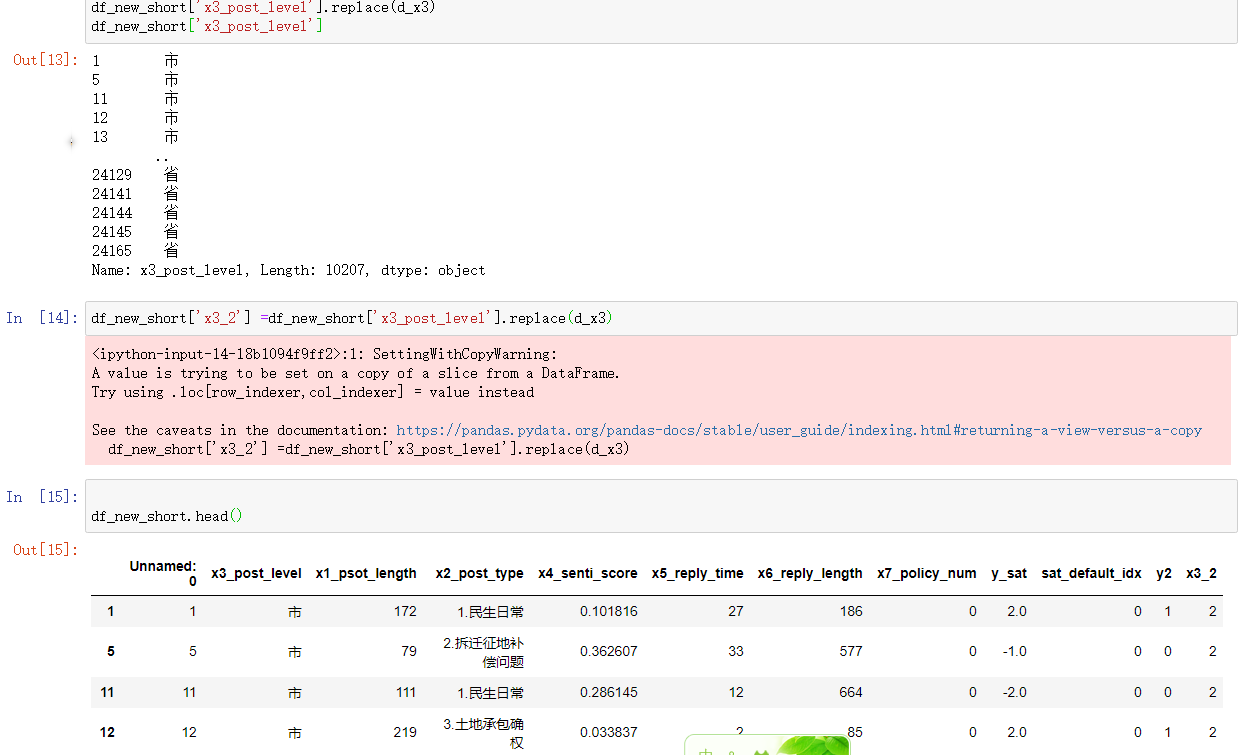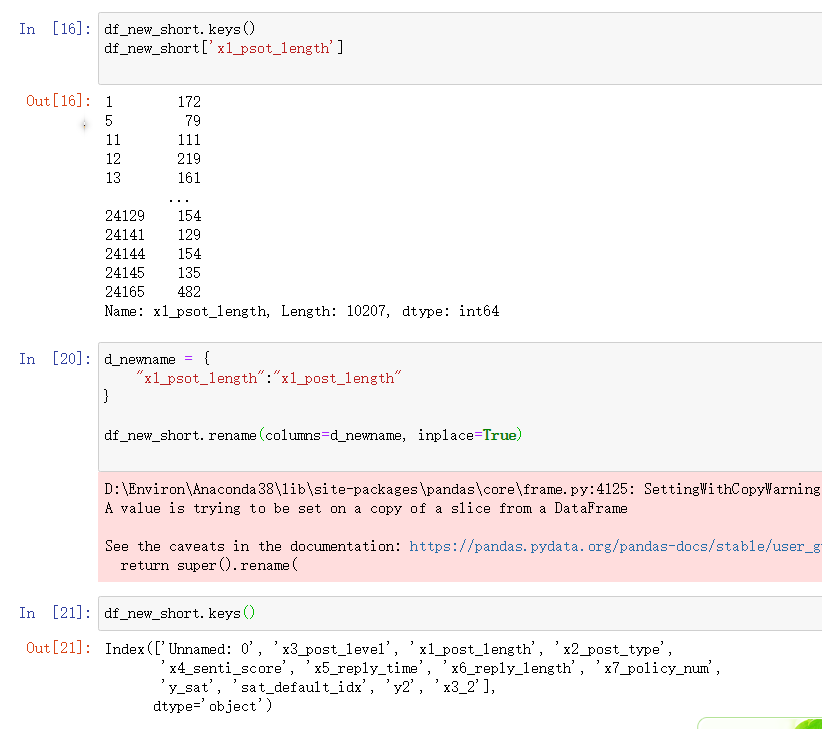• ## python变量赋值

千次阅读 2018-03-05 15:31:55
python2.0开始 等号可以和一个算术操作符组合在一起，将计算结果重新赋值给左边的变量，这被称为增量赋值，类似下面的语句： x=x+1 现在可以被写作： x+=1 增量赋值相对普通赋值不仅仅是写法上的改变，最有...
增量赋值

从python2.0开始 等号可以和一个算术操作符组合在一起，将计算结果重新赋值给左边的变量，这被称为增量赋值，类似下面的语句：

x=x+1
现在可以被写作：
x+=1

增量赋值相对普通赋值不仅仅是写法上的改变，最有意义的变化是第一个对象，金杯处理一次，可变对象会被就地修改（无修拷贝引用），不可变对象则和A=A+B的结果一样（分配一个新的对象）,有一个例外就是A仅被求值一次

>>> m = 12
>>> m % = 7
>>> m
5
>>>m ** = 2
>>>m
25
>>>aList = [123,'xyz']
>>>aList += 
>>>aList
[123.'xyz',456]

Python不支持类似x++或者–x这样的前置/后置自增/自减运算

多重赋值

>>> x = y = z= 1
>>>x
1
>>>y
1
>>>z
1

在上面的例子中，一个值为1的整形对象被创建，该对象的同一个引用被赋值给x,y,z。也就是将一个对象赋给了多个变量，当然，在Python中，将多个对象赋值给多个变量也是可以的。

“多元”赋值

另一种能将多个变量同时赋值的方法我们称为多元赋值（multuple），这不是Python官方术语，而是我们将“mul—tuple”连在一起自创的。因为采用这种方式赋值的时候，等号两边的对象都是tuple

>>>x,y,z = 1,2,'a string'
>>>x
1
>>>y
2
>>>z
'a string'

通常元组需要用圆括号括起来，虽然这是可选的，建议总是加上圆括号一是你的代码有更高的可读性。

**在Python中两个变量交换
x,y=1,2
此时x=1，y=2
然后进行交换
x,y = y,x
此时x=2，y=1
显然，python在赋值之前已经事先对x,y的新值进行了计算**
展开全文• 对数据表的操作经常需要重命名变量，或者中心赋值某个变量，可以使用numpy的where，也可以使用rename， 或者使用replace。 使用字典的方式比较方便。下面是代码： import pandas as pd import statsmodels.api as...
对数据表的操作经常需要重命名变量，或者中心赋值某个变量，可以使用numpy的where，也可以使用rename， 或者使用replace。

使用字典的方式比较方便。下面是代码：

import pandas as pd
import statsmodels.api as sm
import numpy as np
df = pd.read_excel("sn_short_for_reg.xlsx")

df.keys()
df['y_sat']

df_new = df[df['y_sat'].isnull() == False]

df_new.shape

y_sat = df_new['y_sat']
y_sat

y2 = np.where(y_sat >0, 1, 0)

y2
pd.Series(y2).value_counts()

df_new['y2'] = y2

df
df_new

df_new_short = df_new[df_new['sat_default_idx'] == 0]

df_new_short.shape

df_new_short
df_new_short

x3 = df_new_short['x3_post_level']
x3.value_counts()

d_x3 = {
"市":2,
"省":1,
"区":3,
"天津市":1,
"北京市":1,
"自治州":2,
"县":3,
"盟":2,
"重庆市":1,
"上海市":1
}

df_new_short['x3_post_level'].replace(d_x3)
df_new_short['x3_post_level']

df_new_short['x3_2'] =df_new_short['x3_post_level'].replace(d_x3)

df_new_short.head()

df_new_short.keys()
df_new_short['x1_psot_length']

d_newname = {
"x1_psot_length":"x1_post_length"
}

df_new_short.rename(columns=d_newname)

数据表概览

使用where赋值使用字典 对变量重新赋值结果如下：使用字典重命名 变量，要使用inplace选项，不然数据表不会改展开全文• while求1-100 的和 ...在执行到第一行时，i 变量被赋值了1， 而执行到第二行时，先从 i 变量中取出 1 加上 17，然后 i 又被重新赋值成18，再打印 i ，所以最后输出的结果是18。 1 #变量 i 存...

while求1-100 的和
小例子:i = 1i = i + 17print(s)          输出是18原因：赋值是把等号右边的内容放到等号左边的变量里， 而程序是一行一行往下执行的。在执行到第一行时，i 变量被赋值了1， 而执行到第二行时，先从 i 变量中取出 1 加上 17，然后 i 又被重新赋值成18，再打印 i ，所以最后输出的结果是18。

1 #变量 i 存储当前数字 初始值为 1
2 #变量 s 存储当前合计 初始值为 0
3 i = 1
4 s = 0
5 while i <= 100:
6     s += i
7     i += 1
8     print(i,'****',s)
9 '''
10 2 **** 1
11 3 **** 3
12 4 **** 6
13 ...
14 99 **** 4851
15 100 **** 4950
16 101 **** 5050
17 '''

解释输出结果 第一行，
i = 1  s = 0 开始执行程序，（打印的变量是 i +  和 s + ）i += 1 相当于  i = i + 1 = 1 + 1 = 2，则打印结果为  2，s += i 相当于 s = s + i =  0 + 1 = 1，则打印结果为 1，。。。i = 100 时，i += 1 相当于 i = i + 1 = 100 + 1 = 101，则打印结果为101，s += i 相当于 s = s + i =  4950 + 100 = 5050，则打印结果为5050 。

转载于:https://www.cnblogs.com/aston911/p/10042918.html
展开全文• Python 变量赋值 】 while求1-100的和 ...在执行到第一行时，i 变量被赋值了1， 而执行到第二行时，先从 i 变量中取出 1 加上 17，然后 i 又被重新赋值成18，再打印 i ，所以最后输出的结果是18。 #变量 ...
用 while求1-100的和
i = 1
i = i + 17
print(s)          输出是18
原因：
赋值是把等号右边的内容放到等号左边的变量里， 而程序是一行一行往下执行的。在执行到第一行时，i 变量被赋值了1， 而执行到第二行时，先从 i 变量中取出 1 加上 17，然后 i 又被重新赋值成18，再打印 i ，所以最后输出的结果是18。
#变量 i 存储当前数字 初始值为 1
#变量 s 存储当前合计 初始值为 0
i = 1
s = 0
while i <= 100:
s += i
i += 1
print(i,'****',s)

2 **** 1
3 **** 3
4 **** 6
'''
99 **** 4851
100 **** 4950
101 **** 5050

解释输出结果 第一行，
i = 1  s = 0 开始执行程序，（打印的变量是 i +  和 s + ）
i += 1 相当于  i = i + 1 = 1 + 1 = 2，则打印结果为2，
s += i 相当于 s = s + i =  0 + 1 = 1，则打印结果为1，
。。。
i = 100 时，
i += 1 相当于 i = i + 1 = 100 + 1 = 101，则打印结果为101，
s += i 相当于 s = s + i =  4950 + 100 = 5050，则打印结果为5050 。


展开全文基础
• 斐波那契数列的初始子序列，如下所示: a, b = 0, 1 while a < 10: print(a) a, b = b, a+b 这里a,b=b,a+b指的是这体现出...也就是说这里的a+b里面的a的值指的其实是print(a)的a，而不是下方被重新赋值的a ...
• python中，形如X = Y的拷贝都是浅拷贝，X和Y是公用同一块空间的，一旦对它们其中的任意一个进行数据操作，都会改变该空间的内容，除非重新赋一块空间，改变其指向的位置。 因此只需要改成： X = [] Y = [] 就可以...
• 赋值语句包含三个主要部分：①左值、②赋值运算符、③右值 赋值语句有两个用途：①定义新的变量。②让已定义的变量指向特定值 ...第二条赋值语句x=9为x重新赋值。让它指向另外一个值9.它没有创建变量。因为x这个变量
• 今天去刷leetcode，遇到一道题： ...  我的方法是先把matrix赋值给一个新的变量，直接写两个for循环直接把matrix...然后我惊奇地发现，我修改（注意是修改不是重新赋值，就是.append()操作）新变量，而matrix同时也...
• python字典的重新赋值，例如 temp = {"a":'b'} temp = {'a','c'} 是重新建立了一个新的字典，temp的id改变 如下代码可以体现 comments = [] temp = {"a":'b'} print(id(temp)) comments.append(temp) print...
• Python中最基本的内置数据类型：整型、浮点型、布尔型（True、False）、字符串型（“**”） 使用divmod()函数同时得到商和余数。如输入：divmod(13, 3)，则输出(4, 1)。 数字： 正在上传…重新上传取消 正在...机器学习
• 关于python中列表、字典的深浅拷贝问题之前认识比较模糊，之前的笔记中其实只是区分了拷贝和赋值的问题， 今天以列表为例重新学习一下： 关于深浅拷贝和赋值的概念： a.直接赋值： 用等号 = ，其实就是对象的引用...list copy 深拷贝 浅拷贝
• 重新赋值时，又会重新开辟内存空间然后将新的数据和变量相关联，并且删除变量之前关联的数据。 运算符： python中的运算符：数学运算符、比较运算符、逻辑运算符和赋值运算符 数学运算符：+、 -、 *、 /、 %
• Python :h5py 如何对dataset进行重新赋值？ 建议先删除原有的dataset,再进行重新赋值。 f = h5py.File(“temp.h5”,“a”) g =f.create_group("\data") g.create_dataset(“ib”,data =data) g[“ib”] = g...
• 现在给变量a重新赋值 a = 2; 盒子依然是那个盒子，也就是说内存地址没有变，只是该段内存中的值变了，变成了2。 再来看： int b = a; 当把变量a赋值给另外一个变量b时，相当于把值拷贝了一份传递给变量b，b是新...
• Python是一门独特的语言，与C语言有很大区别，初学Python很多萌新表示对变量与赋值不理解，学过C的都知道，给变量赋值时，需要先指定数据类型，...现在给变量a重新赋值 a = 2; 盒子依然是那个盒子，也就是说内...
• deepcopy: 在内存中重新创建所有子元素 自定义 deepcopy: my_deepcopy = lambda item: loads(dumps(item, 4)) 练习：说出执行结果 def extendList(val, lst=[]): lst.append(val) return lst.
• Python是一门独特的语言，与C语言有很大区别，初学Python很多萌新表示对变量与赋值不理解，学过C的都知道，给变量赋值时，...现在给变量a重新赋值a = 2; 盒子依然是那个盒子，也就是说内存地址没有变，只是该段内存...变量
• 注意: 不是表示不可以修改变量的值 而是表示:重新赋值，实际上是重新创建一个不可变类型的对象,　原对象如果有引用, 就还是原有的值 如果没有引用就被回收 Python的每个对象都分为可变和不可变，主要的核心类型中，...
• 赋值为5， 4， 3， 2， 1 方法如下： 定义一个字典 y_dict = { "很满意":5, "满意":4, "基本满意":3, "不满意":2, "很不满意":1 } 然后使用map，这里‘y_sat'是原来的变量，即字符串格式的变量，’y...字符串
• python中定义变量和重新给变量赋值的原理： 当给变量赋值的时候，会先在内存中开辟空间保持数据(开辟的内存空间的大小由数据决定 然后数据对应的内存空间和变量进行关联。重新给变量赋值的时候，优惠重新开辟新的...
• 在可变类型中，我们可以修改变量的赋值而不改变数据的内存地址，而在不可变类型中，我们想要修改变量的赋值，必须重新赋值， 如以下demo 字符串： >>> str = "1234" >>> str_01...
• 而copy_a,deepcopy_a与a的地址都不一致，说明copy_a,deepcopy_a是重新开辟了地址空间存放新的对象。 所以，对b的操作等同于对a的操作，而无论是深度拷贝还是浅度拷贝，对新对象的操作不会影响到原对象（对象不...
• 前面讲了python的可变对象和不可变对象，总结起来就是，可变对象指向的内存中的值可以修改，不可变对象指向的内存的中的值不可以修改，如果想改变这个对象的值，会重新申请一个内存地址，修改值后存到新的地址上，...拷贝...

# python重新赋值python 订阅